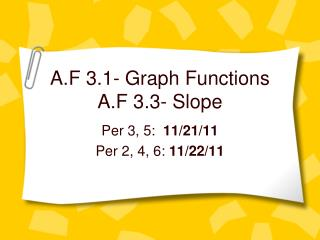DownloadDownload PresentationA.F 3.1- Graph Functions A.F 3.3- Slope

A.F 3.1- Graph Functions A.F 3.3- Slope

Download PresentationA.F 3.1- Graph Functions A.F 3.3- Slope

- - - - - - - - - - - - - - - - - - - - - - - - - - - E N D - - - - - - - - - - - - - - - - - - - - - - - - - - -
Presentation Transcript

1. A.F 3.1- Graph FunctionsA.F 3.3- Slope Per 3, 5: 11/21/11 Per 2, 4, 6: 11/22/11

2. AF 3.1- Graph Functions • You will be given a graph and must select the equation that best matches that graph.

3. Graph Equations • y= x straight line (pointing right) • y= -x straight line (pointing left) • y= x2parabola (U shape, open up) • y= -x2 parabola (U shape, open down) • y= x3 cubic graph (snake shape, twisted) • y= -x3 cubic graph (snake shape, twisted)

4. AF 3.3- Slope • The slope is the number that tells how much a line rises (goes up) or falls (goes down) as it moves from left to right across the x-y plane.

5. Slope Equation • With the slope equation, you can figure out the slope between any two ordered pairs (x1, y1) and (x2, y2). • Slope= rise= y2-y1 run x2-x1

6. Example • Here’s how you would use the slope equation to find slope between the ordered pairs (-3, -2) and (1, 6): • Step 1: The first ordered pair is your (x1, y1), so: (x1, y1)= (-3, -2) x1= -3 y1= -2

7. Step 2: • The second ordered pair is your (x2, y2), so: (x2, y2)= (1,6) x2= 1 y2=6

8. Step 3: • Plug the values where they go into the slope equation, and solve: Slope= y2-y1= 6-(-2)= 6+2 = 8 x2-x1 1-(-3) 1+3 4 =2

9. Slope cont. • BIG NOTE: If you are asked to figure out the slope of a line, • Pick any two points on the line and • plug their coordinates into the slope formula.

10. Example: Given this graph: Find the slope

11. Step 1: • Pick any two points. We’ll select (-2, -3) The ordered pair of any first point is your (x1, y1)

12. Step 2 • The second ordered pair is your (x2, y2): We’ll select (4,0)

13. Step 3: • Plug the values into the slope equation: Slope= y2-y1 x2-x1 Finish the problem and box your answer